# Identify position and number to find the odd one out

In this worksheet, students will identify an image that does not fit in with the rest of the group. The focus will be on position and number, so students will need to look at where shapes are and how many there are.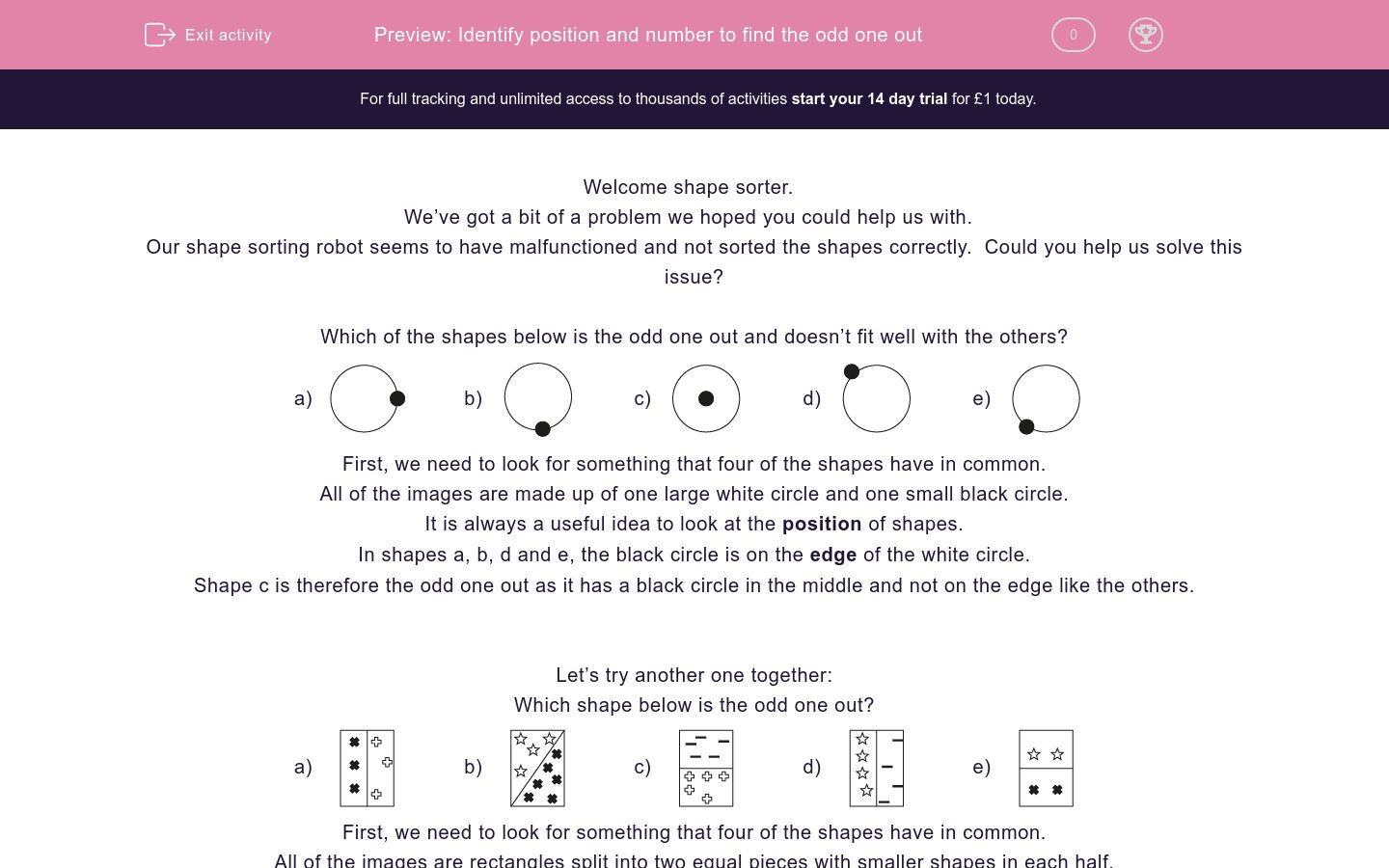Key stage:  KS 2

Curriculum topic:   Spatial and Non-Verbal Reasoning

Curriculum subtopic:   Odd One Out

Difficulty level:### QUESTION 1 of 10

Welcome shape sorter.

We’ve got a bit of a problem we hoped you could help us with.

Our shape sorting robot seems to have malfunctioned and not sorted the shapes correctly.  Could you help us solve this issue?

Which of the shapes below is the odd one out and doesn’t fit well with the others?

a)b)c)d)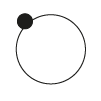e)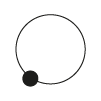First, we need to look for something that four of the shapes have in common.

All of the images are made up of one large white circle and one small black circle.

It is always a useful idea to look at the position of shapes.

In shapes a, b, d and e, the black circle is on the edge of the white circle.

Shape c is therefore the odd one out as it has a black circle in the middle and not on the edge like the others.

Let’s try another one together:

Which shape below is the odd one out?

a)b)c)d)e)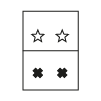First, we need to look for something that four of the shapes have in common.

All of the images are rectangles split into two equal pieces with smaller shapes in each half.

In questions like this, counting is really important. What could we count?

Shape a has 3 shapes on each side of the halfway line.

Shape b has 4 shapes on one side and 6 on the other.

Shape c has 5 shapes on each side of the line.

Shape d has 4 shapes on either side.

Shape e has 2 shapes on either side.

This means that image b is the odd one out as it has a different number of shapes in each section, unlike the other options.

It’s now your turn to identify the odd shape.

Remember to look at the position of shapes within the image and always see if there is something you can count.

Good luck shape sorter!

Which of the images below is the odd one out and doesn't fit well with the others?

a)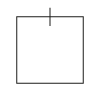b)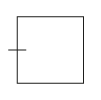c)d)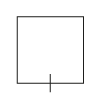e)Which of the images below is the odd one out and doesn't fit well with the others?

a)b)c)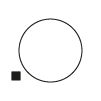d)e)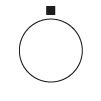Which of the images below is the odd one out and doesn't fit well with the others?

a)b)c)d)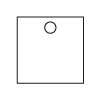e)Which of the images below is the odd one out and doesn't fit well with the others?

a)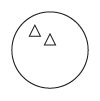b)c)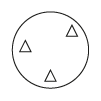d)e)Which of the images below is the odd one out and doesn't fit well with the others?

a)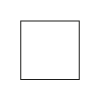b)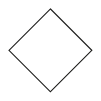c)d)e)Which of the images below is the odd one out and doesn't fit well with the others?

a)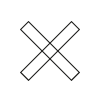b)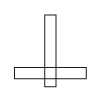c)d)e)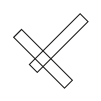Which of the images below is the odd one out and doesn't fit well with the others?

a)b)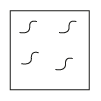c)d)e)Which of the images below is the odd one out and doesn't fit well with the others?

a)b)c)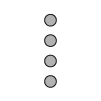d)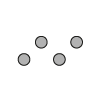e)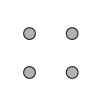Which of the images below is the odd one out and doesn't fit well with the others?

a)b)c)d)e)Which of the images below is the odd one out and doesn't fit well with the others?

a)b)c)d)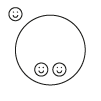e)• Question 1

Which of the images below is the odd one out and doesn't fit well with the others?

e)EDDIE SAYS
This question focuses on position. In the first four options, the small line is positioned on the edge of the square. In the last option, the line is inside the square. This makes option e the odd one out as the position of its small line doesn't match the others.
• Question 2

Which of the images below is the odd one out and doesn't fit well with the others?

d)EDDIE SAYS
Here is another position-based question. In options a, b, c and e the black square is outside the circle. In option d, the square is in the middle of the circle. This makes option d the odd one out.
• Question 3

Which of the images below is the odd one out and doesn't fit well with the others?

c)EDDIE SAYS
I hope you have your position hat on! Four of these images have small squares positioned at the top of the outer square. Option c is the odd one out, as its small shape is positioned in the middle of the square, rather than at the top.
• Question 4

Which of the images below is the odd one out and doesn't fit well with the others?

c)EDDIE SAYS
This question focuses on number. What can we count in these images? In options a, b, d and e there are two triangles inside each triangle, whereas option c has three triangles. How greedy! This makes option c the odd one out.
• Question 5

Which of the images below is the odd one out and doesn't fit well with the others?

d)EDDIE SAYS
It's time to count again! Here we have to count the number of sides that each shape has. Options a, b, c and e all have four sides, but option d only has three. This makes option d the odd triangle out.
• Question 6

Which of the images below is the odd one out and doesn't fit well with the others?

d)EDDIE SAYS
What can we count here? Options a, b, c and e are all made up of two overlapping rectangles. Option d has three! This makes option d the odd one out.
• Question 7

Which of the images below is the odd one out and doesn't fit well with the others?

b)EDDIE SAYS
Be careful with this one detective! In this question, the outside shapes are all different. This is a big clue that we have to look inside the shapes to spot the clue which will enable us to find the correct answer. Options a, c, d and e all have three squiggly lines inside of them, but option b has four. This means that option b is the odd one out.
• Question 8

Which of the images below is the odd one out and doesn't fit well with the others?

a)EDDIE SAYS
Here's another one that you need to make COUNT. The positions of the circles are all different so this doesn't help us out. Option a has only 3 circles, but the other four options have 4 circles. This means that option a is the odd one out.
• Question 9

Which of the images below is the odd one out and doesn't fit well with the others?

d)EDDIE SAYS
We have some overlapping shapes here to really test our detectives' eyes! You may notice that in options a, b, c and e the square overlaps the rectangle in a corner, but in option d the square overlaps the middle of the rectangle, rather than in a corner. This makes option d the odd one out.
• Question 10

Which of the images below is the odd one out and doesn't fit well with the others?

b)EDDIE SAYS
Get ready for a double challenge here top detective! This question involves both position and number. We have to count the number of smiley faces and look at where they are, too. In options a, c, d and e there are only 2 smiley faces on the inside of the circle and 1 on the outside. Option b has 1 smiley face on the inside and 1 on the outside, so this must be the odd one out. Great clue spotting there detective - well done!
---- OR ----